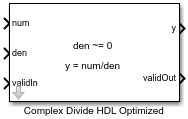# Complex Divide HDL Optimized

Divide one input by another and generate optimized HDL code

• Library:
• Fixed-Point Designer HDL Support / Math Operations

•## Description

The Complex Divide HDL Optimized block outputs the result of dividing the scalar num by the scalar den, such that y = num/den.

## Limitations

Data type override is not supported for the Complex Divide HDL Optimized block.

## Ports

### Input

expand all

Numerator, specified as a scalar.

Data Types: `single` | `double` | `fixed point`
Complex Number Support: Yes

Denominator, specified as a scalar.

Data Types: `single` | `double` | `fixed point`
Complex Number Support: Yes

Whether input is valid, specified as a Boolean scalar. This control signal indicates when the data from the num and den input ports are valid. When this value is `1` (`true`), the block captures the values at the input ports num and den. When this value is `0` (`false`), the block ignores the input samples.

Data Types: `Boolean`

### Output

expand all

Output computed by dividing num by den, such that y = num/den, returned as a complex scalar with data type specified by Output datatype.

Data Types: `single` | `double` | `fixed point`

Whether the output data is valid, returned as a Boolean scalar. When the value of this control signal is `1` (`true`), the block has successfully computed the output at port y. When this value is `0` (`false`), the output data is not valid.

Data Types: `Boolean`

## Parameters

expand all

Data type of output y, specified as `fixdt(1,18,10)`, `single`, `fixdt(1,16,0)`, or as a user-specified data type expression. The type can be specified directly or expressed as a data type object, such as `Simulink.NumericType`.

#### Programmatic Use

 Block Parameter: `OutputType` Type: character vector Values: `'fixdt(1,18,10)'` | `'single'` | `'fixdt(1,16,0)'` | ```''``` Default: `'fixdt(1,18,10)'`

## Algorithms

For fixed-point inputs num and den, the Complex Divide HDL Optimized block wraps on overflow for division by zero. The behavior for fixed-point division by zero is summarized in the table below.

Wrap OverflowSaturate Overflow
0/0 = 00/0 = 0
1/0 = 01/0 = upper bound
-1/0 = 0-1/0 = lower bound

For floating-point inputs, the Complex Divide HDL Optimized block follows IEEE® Standard 754.

## Extended Capabilities